Mathematics
Easy

Question

# Which of the following represents the graph?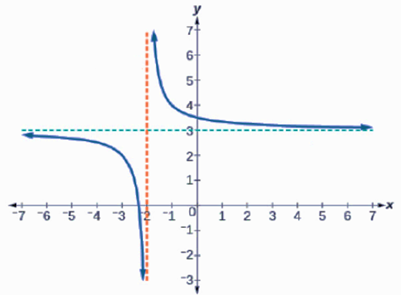## f(x) =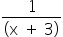– 2 f(x) =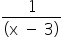+ 2f(x) =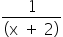+ 3f(x) =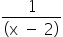+ 3Hint:

## The correct answer is: f(x) =+ 3

### Here, we have to find the equation of the given graph.Here f(x) =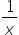has shifted 3 units up and 2 units to the left. 3 units up means: output (f(x)) increased by 3 units.2 units to the left: input (x) increased by 2 units.So, f(x) =+ 3.Hence, the correct option is C.

There are many ways to transform a graph such as vertical translation, vertical stretch, horizontal translation and horizontal stretch.

### Related Questions to study#### With Turito Foundation.#### Get an Expert Advice From Turito.